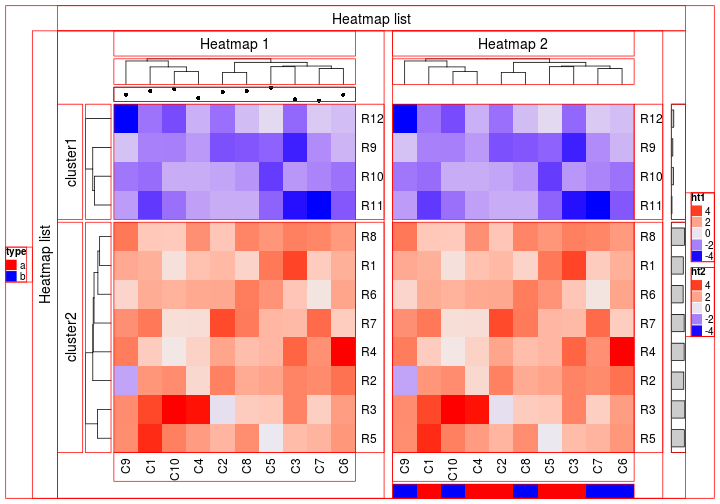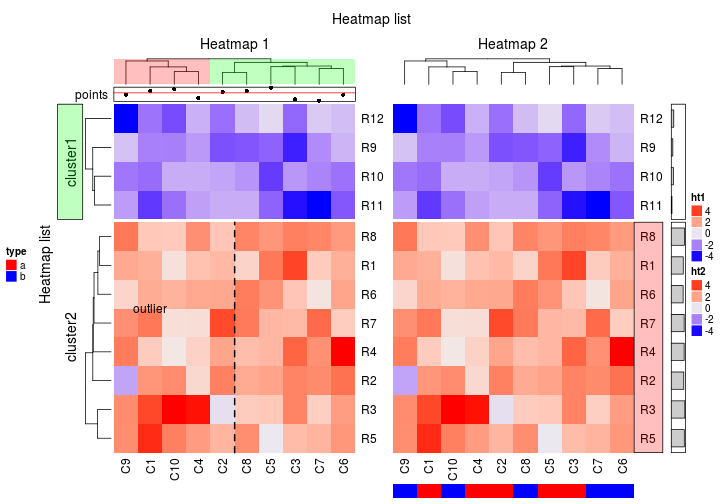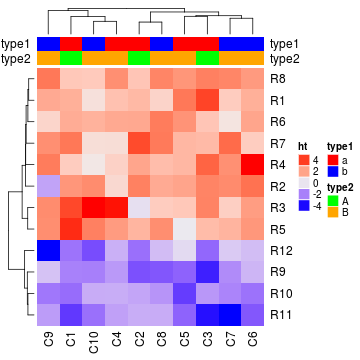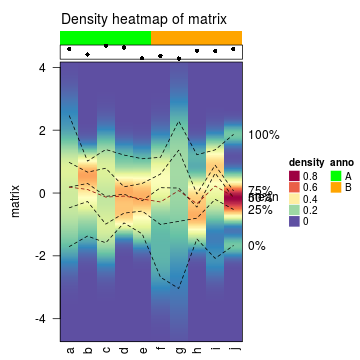# Heatmap Decoration

Author: Zuguang Gu ( z.gu@dkfz.de )

Date: 2017-04-24

Each components of the heatmap/heatmap list has a name (unique id). You can go to any viewport to add graphics in by specifying the heatmap/annotation name.

First generate a figure that almost contains all types of heatmap components.

``````library(ComplexHeatmap)

mat = matrix(rnorm(80, 2), 8, 10)
mat = rbind(mat, matrix(rnorm(40, -2), 4, 10))
rownames(mat) = paste0("R", 1:12)
colnames(mat) = paste0("C", 1:10)

ha_column1 = HeatmapAnnotation(points = anno_points(rnorm(10)))
ht1 = Heatmap(mat, name = "ht1", km = 2, row_title = "Heatmap 1", column_title = "Heatmap 1",
top_annotation = ha_column1)

ha_column2 = HeatmapAnnotation(df = data.frame(type = c(rep("a", 5), rep("b", 5))),
col = list(type = c("a" = "red", "b" = "blue")))
ht2 = Heatmap(mat, name = "ht2", row_title = "Heatmap 2", column_title = "Heatmap 2",
bottom_annotation = ha_column2)
``````The components (viewports) that have names are:

• `global`: the viewport which contains the whole figure.
• `global_column_title`: the viewport which contains column title for the heatmap list.
• `global_row_title`: the viewport which contains row title for the heatmap list.
• `main_heatmap_list`: the viewport which contains a list of heatmaps and row annotations.
• `heatmap_@{heatmap_name}`: the viewport which contains a single heatmap
• `annotation_@{annotation_name}`: the viewport which contains an annotation on columns.
• `annotation_@{annotation_name}_@{i}`: for row annotations
• `@{heatmap_name}_heatmap_body_@{i}`: the heatmap body.
• `@{heatmap_name}_column_title`: column title for a single heatmap.
• `@{heatmap_name}_row_title_@{i}`: since a heatmap body may be splitted into several parts. `@{i}` is the index of the row slice.
• `@{heatmap_name}_dend_row_@{i}`: dendrogram for ith row slice.
• `@{heatmap_name}_dend_column`: dendrogram on columns
• `@{heatmap_name}_row_names_@{i}`: the viewport which contains row names.
• `@{heatmap_name}_column_names`: the viewport which contains column names.
• `heatmap_legend`: the viewport which contains all heatmap legends.
• `legend_@{heatmap_name}`: the viewport which contains a single heatmap legend.
• `annotation_legend`: the viewport which contains all annotation legends.
• `legend_@{annotation_name}`: the viewport which contains a single annotation legend.

## decorate_* functions

Basically, you can go to these components by `seekViewport()`, but to hide the details that is too low-level, ComplexHeatmap package provides `decorate_*` family functions which makes it easy to add graphics into different components.

Following code add annotation names, mark one grid in the heatmap and seperate the first column clusters with two rectangles.

``````ht_list = draw(ht_list, row_title = "Heatmap list", column_title = "Heatmap list",
heatmap_legend_side = "right", annotation_legend_side = "left")
decorate_annotation("points", {
grid.text("points", unit(0, "npc") - unit(2, "mm"), 0.5,
default.units = "npc", just = "right")
})

decorate_heatmap_body("ht1", {
grid.text("outlier", 1.5/10, 2.5/4, default.units = "npc")
grid.lines(c(0.5, 0.5), c(0, 1), gp = gpar(lty = 2, lwd = 2))
}, slice = 2)

decorate_column_dend("ht1", {
tree = column_dend(ht_list)\$ht1
ind = cutree(as.hclust(tree), k = 2)[order.dendrogram(tree)]

first_index = function(l) which(l)
last_index = function(l) { x = which(l); x[length(x)] }
x1 = c(first_index(ind == 1), first_index(ind == 2)) - 1
x2 = c(last_index(ind == 1), last_index(ind == 2))
grid.rect(x = x1/length(ind), width = (x2 - x1)/length(ind), just = "left",
default.units = "npc", gp = gpar(fill = c("#FF000040", "#00FF0040"), col = NA))
})

decorate_row_names("ht2", {
grid.rect(gp = gpar(fill = "#FF000040"))
}, slice = 2)

decorate_row_title("ht1", {
grid.rect(gp = gpar(fill = "#00FF0040"))
}, slice = 1)

decorate_annotation("points", {
grid.lines(c(0, 1), unit(c(0, 0), "native"), gp = gpar(col = "red"))
})
``````For annotations which are created by `anno_points()`, `anno_barplot()` and `anno_boxplot()`, “native” unit can be used in the decoration code.

By default, annotation names are not plotted along with the heatmap annotations. The reason is if annotation names is plotted, they will located in the area of other heatmap components which would makes the adjustment of the heatmap layout difficult. `HeatmapAnnotation()` provides a not-so-perfect solution for adding annotation names, however, since you can go to any component in the heatmap list by its name, actually it is not difficult to add annotation names manually.

Following code add annotation names on the both sides of the column annotations. The drawback is since there is no specific component designed for annotation names, if the annotation name is too long, it will be exceeding the figure region (but this problem can be solved by some tricks, see the Examples vignette).

``````df = data.frame(type1 = c(rep("a", 5), rep("b", 5)),
type2 = c(rep("A", 3), rep("B", 7)))
ha = HeatmapAnnotation(df, col = list(type1 = c("a" = "red", "b" = "blue"),
type2 = c("A" = "green", "B" = "orange")))
Heatmap(mat, name = "ht", top_annotation = ha)
for(an in colnames(df)) {
decorate_annotation(an, {
# annotation names on the right
grid.text(an, unit(1, "npc") + unit(2, "mm"), 0.5, default.units = "npc", just = "left")
# annotation names on the left
grid.text(an, unit(0, "npc") - unit(2, "mm"), 0.5, default.units = "npc", just = "right")
})
}
``````## Visualize distributions

With heatmap decorations, actually you can design new graphics based on heatmaps. Following is an example:

To visualize distribution of columns in a matrix or in a list, sometimes we use boxplot or beanplot. Here we can also use colors to map to the density values and visualize distribution of values in each column (or each list element) through a heatmap. Sometimes it can give you a more clear image of your data.

Here the package has a `densityHeatmap()` function, the usage is quite straightforward:

``````matrix = matrix(rnorm(100), 10); colnames(matrix) = letters[1:10]
ha = HeatmapAnnotation(df = data.frame(anno = rep(c("A", "B"), each = 5)),
col = list(anno = c("A" = "green", "B" = "orange")),
points = anno_points(runif(10)))
densityHeatmap(matrix, anno = ha)
``````## Session info

``````sessionInfo()
``````
``````## R version 3.4.0 (2017-04-21)
## Platform: x86_64-pc-linux-gnu (64-bit)
## Running under: Ubuntu 16.04.2 LTS
##
## Matrix products: default
## BLAS: /home/biocbuild/bbs-3.5-bioc/R/lib/libRblas.so
## LAPACK: /home/biocbuild/bbs-3.5-bioc/R/lib/libRlapack.so
##
## locale:
##   LC_CTYPE=en_US.UTF-8       LC_NUMERIC=C               LC_TIME=en_US.UTF-8
##   LC_COLLATE=C               LC_MONETARY=en_US.UTF-8    LC_MESSAGES=en_US.UTF-8
##  LC_TELEPHONE=C             LC_MEASUREMENT=en_US.UTF-8 LC_IDENTIFICATION=C
##
## attached base packages:
##   stats4    parallel  grid      stats     graphics  grDevices utils     datasets  methods
##  base
##
## other attached packages:
##   GetoptLong_0.1.6      dendextend_1.5.2      dendsort_0.3.3        cluster_2.0.6
##   HilbertCurve_1.6.0    GenomicRanges_1.28.0  GenomeInfoDb_1.12.0   IRanges_2.10.0
##   S4Vectors_0.14.0      BiocGenerics_0.22.0   circlize_0.3.10       ComplexHeatmap_1.14.0
##  knitr_1.15.1          markdown_0.8
##
## loaded via a namespace (and not attached):
##   fastcluster_1.1.22      shape_1.4.2             modeltools_0.2-21       kernlab_0.9-25
##   lattice_0.20-35         colorspace_1.3-2        viridisLite_0.2.0       prabclus_2.2-6
##   RColorBrewer_1.1-2      fpc_2.1-10              GenomeInfoDbData_0.99.0 plyr_1.8.4
##  robustbase_0.92-7       stringr_1.2.0           zlibbioc_1.22.0         munsell_0.4.3
##  gtable_0.2.0            GlobalOptions_0.0.11    mvtnorm_1.0-6           evaluate_0.10
##  flexmix_2.3-13          class_7.3-14            highr_0.6               DEoptimR_1.0-8
##  trimcluster_0.1-2       Rcpp_0.12.10            scales_0.4.1            diptest_0.75-7
##  XVector_0.16.0          mime_0.5                gridExtra_2.2.1         rjson_0.2.15
##  ggplot2_2.2.1           png_0.1-7               stringi_1.1.5           tools_3.4.0
##  bitops_1.0-6            HilbertVis_1.34.0       magrittr_1.5            lazyeval_0.2.0
##  RCurl_1.95-4.8          tibble_1.3.0            whisker_0.3-2           MASS_7.3-47
##  viridis_0.4.0           mclust_5.2.3            nnet_7.3-12             compiler_3.4.0
``````